# Complexation of NpO2+ with (2-hydroxyethyl)ethylenediaminetriacetic acid (HEDTA) in aqueous solutions: thermodynamic studies and structural analysis†

Xingliang Liab, Zhicheng Zhang*a, Leigh R. Martinc, Shunzhong Luo*b and Linfeng Raoa
aChemical Sciences Division, Lawrence Berkeley National Laboratory, Berkeley, California 94720, USA. E-mail: lxzhang@lbl.gov; Fax: +1 5104865596; Tel: +1 5104865141
bInstitute of Nuclear Physics and Chemistry, China Academy of Engineering Physics, Mianyang, Sichuan 621999, China. E-mail: luoshzh@caep.cn
cAqueous Separations and Radiochemistry Department, Idaho National Laboratory, PO Box 1625, Idaho Falls, ID 83415, USA

Received 12th July 2016 , Accepted 1st December 2016

First published on 2nd December 2016

Complexation of Np(V) with N-(2-hydroxyethyl)ethylenediaminetriacetic acid (HEDTA) was studied in aqueous solution (I = 1.0 mol L−1 NaClO4, t = 25 °C) by spectrophotometry, microcalorimetry and Extended X-ray absorption fine structure (EXAFS) spectroscopy. Equilibrium constants for the formation of three complexes, NpO2L2−, NpO2(HL), and (NpO2)2(OH)2L26−, were determined to be (6.91 ± 0.06), (4.28 ± 0.03) and −(4.93 ± 0.03), respectively. The enthalpies of complexation were determined to be −(8.0 ± 2.0) kJ mol−1 for NpO2L2− and −(2.2 ± 2.0) kJ mol−1 for NpO2(HL). Thermodynamic data of the complexation of Np(V) with HEDTA were compared to those of Np(V) with other aminopolycarboxylic acids, gaining insight into the possible coordination modes of the complexes. The EXAFS studies provided further structural information on those modes. In both NpO2L2− and NpO2(HL) complexes, HEDTA coordinates to Np(V) in a tridentate mode through two oxygens of two carboxylic groups and one nitrogen of the amine group. In the (NpO2)2(OH)2L26− complex, two Np(V) atoms are bridged by two hydroxides and each HEDTA maintains the tridentate coordination mode.

## 1 Introduction

Large quantities of high-level nuclear waste (HLW), generated by the weapons program in the United States, are currently stored in single-shelled or double-shelled storage tanks on Department of Energy (DOE) sites such as the Hanford site in the state of Washington. Some of these waste tanks have either leaked or been overfilled, resulting in the release of radioactive contaminants into the surrounding soils and sediments that raises significant environmental concerns.1 The current strategy for safe management of this type of HLW includes the vitrification and disposal in a geological repository. In the repository, the engineered barrier systems that are installed to isolate the waste forms from the environment may gradually deteriorate and eventually lose integrity. As a result, ground water could get into contact with the waste, dissolve and carry radionuclides into the surrounding geological environment. It is clear that a fundamental understanding of the interactions between the radionuclides and the major chemical components in the wastes is critical for safely managing the HLWs in the repository and the surrounding environment.

The composition of nuclear wastes is extremely complicated and diverse. Besides the radioactive contaminants (90Sr, 137Cs, 99Tc, 129I, lanthanides, 235/238U, and transuranic elements, etc.), varieties of non-radioactive inorganic constituents (Na+, Cl, NO3, CO32−, PO43−, and SO42−, etc.) and organic chemicals also exist.2–8 Among those organic chemicals, a number of carboxylic acids are of great concern because of their complexing capacities. According to the inventory of the Waste Isolation Pilot Plant (WIPP, New Mexico, USA), significant amounts of (2-hydroxyethyl)ethylenediaminetriacetic acid (HEDTA, Fig. 1) are present in the wastes on the DOE sites.5,7,8 For example, the initial concentration of HEDTA in some HLW tanks at Hanford is as high as 0.2 mol L−1 and the total amount of HEDTA from the Hanford mixed waste alone is about 1500 tons.8 HEDTA is expected to form strong complexes with actinides and significantly increase the solubility of actinides in aquatic systems which could in turn escalate their migration in the environment.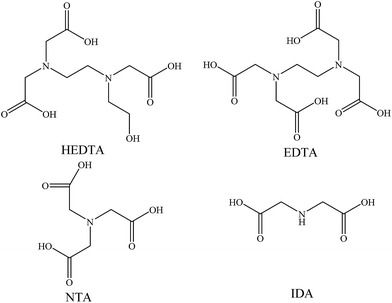Fig. 1 HEDTA and aminopolycarboxylic acids for comparison. HEDTA: (2-hydroxyethyl)ethylenediaminetriacetic acid; EDTA: ethylenediaminetetraacetic acid; NTA: nitrilotriacetic acid; IDA: iminodiacetic acid.

Besides being an important complexant in nuclear wastes, HEDTA has recently been considered a candidate ligand for actinide separations.9 Systematic thermodynamic studies are needed to evaluate the complexation of HEDTA with lanthanides and actinides in the separation processes. In comparison with other aminopolycarboxylic acids,10–14 such as EDTA and NTA, fewer studies of HEDTA have been conducted and thermodynamic data are scarce. In the literature, there have been only two studies15,16 that report thermodynamic data for the complexation of HEDTA with Np(V), a radionuclide which could be problematic in the separation processes and environmental transportation. In a most recent study, Thakur et al. studied the complexation of Np(V) with HEDTA in a pH range of 5.0 to 5.5 by solvent extraction. Due to the narrow pH range of this work, the solvent extraction data were fitted with the model including only one Np(V)/HEDTA complex, i.e., NpO2L2− (where L3− stands for the fully deprotonated HEDTA ligand), and the stability constant (logβ101) was determined to be (7.25 ± 0.06) at 25 °C and I = 6.6 molality NaClO4.16 A few decades earlier, Eberle et al. studied the complexation of Np(V) with HEDTA by spectrophotometry in a pH range of 3–12 and fitted the data with a model including NpO2L2−, NpO2(HL), and NpO2(OH)L3−.15 The stability constants of the three complexes (denoted as logβ101, logβ1(11), and logβ1−11) were determined to be (6.87 ± 0.11), (4.06 ± 0.01), and (11.37 ± 0.10), respectively, at 25 °C and I = 0.1 mol L−1 (Na/NH4)ClO4. The stability constants for the NpO2L2− complex (logβ101) from the two studies, (7.25 ± 0.06) at I = 6.6 m and (6.87 ± 0.11) at I = 0.1 mol L−1, seem to be in reasonably good agreement, taking into consideration the significant difference in the ionic strength. However, the stability constants for NpO2(HL) and NpO2(OH)L3− species need additional scrutiny, because neither the spectra nor the details on the fitting are provided in the publication to allow the evaluation of the quality of the stability constants.

Besides the stability constants, the enthalpy of complexation for the Np(V)/HEDTA complex(es) is another thermodynamic parameter that is critically important to the understanding or prediction of the chemical behavior of Np(V) in the processes involving the use of HEDTA, as separation processes are usually operated at temperatures above 25 °C. No enthalpies of complexation were determined in the above-mentioned two studies.15,16

Due to the uncertainty on the complexation model and associated stability constants and the absence of data on the enthalpy of complexation for the Np(V)/HEDTA system in the literature, spectrophotometric and calorimetric experiments on the complexation of Np(V) with HEDTA were conducted at I = 1.0 mol L−1 NaClO4 in a wide pH range to determine the stability constants and enthalpy of complexation in the present study. Also, X-ray absorption spectroscopy (XAS) was used to investigate the coordination modes in the Np(V)/HEDTA complexes. The stability constants and coordination modes in the Np(V)/HEDTA complexes are discussed in comparison with Np(V) complexes with other aminopolycarboxylic acids.

## 2 Experimental

### 2.1 Chemicals

All chemicals were reagent grade or higher. Milli-Q water was boiled for removing carbon dioxide and then used for the preparation of all solutions. All experiments were conducted at 25 °C and an ionic strength of 1.0 mol L−1 (NaClO4).

The stock solution of Np(V) in HClO4 was prepared as follows. Solid neptunium dioxide (NpO2) was dissolved in concentrated HCl under low heating. Concentrated HClO4 was added to drive away the excess HCl and oxidize neptunium to Np(VI) by fuming. Into the pink solution of Np(VI), a small amount of NaNO2 was added to reduce Np(VI) to Np(V), resulting in a green solution. The Np(V) was then precipitated as hydroxide with 1.0 mol L−1 NaOH. The precipitates were washed three times with deionized water to ensure the removal of excess NaOH and then dissolved with 1.0 mol L−1 HClO4. Absorption spectra were collected to confirm that Np(V) was the only oxidation state in the stock solution. The concentration of Np(V) was determined by the absorbance at 980.2 nm (using the molar absorptivity of 395 L mol−1 cm−1).17 Gran's titration method was used to determine the concentration of free acid in the Np(V) stock solution.18

Stock solutions of HEDTA and NTA were prepared by dissolving in water desired amounts of HEDTA (Sigma-Aldrich) or NTA (J. T. Baker). Working solutions of HEDTA and NTA were prepared by diluting the stock solutions and adding appropriate amounts of HClO4 or NaOH. The ionic strength of all working solutions was adjusted to 1.0 mol L−1 (NaClO4) by adding appropriate quantities of a concentrated NaClO4 solution.

### 2.2 Spectrophotometric titrations

Near-IR absorption spectra of the NpO2+/HEDTA and NpO2+/NTA systems were collected (from 1150 to 950 nm, 0.2 nm interval) on a Cary 6000i spectrophotometer (Varian Inc.). The sample compartment of the instrument was constantly purged with N2 during the experiments. The temperature of the sample and reference cells was maintained at 25 °C by a Peltier device coupled with a circulating water bath. Two types of titrations were conducted for the Np(V)/HEDTA system: in one type, HEDTA was added into the cuvette solution containing Np(V); in the other, NaOH was added into the cuvette solution containing both Np(V) and HEDTA. Multiple titrations with different concentrations of NpO2+, H+, and HEDTA or NTA (CNp, CH, and CL) were performed. The data were analyzed with the HypSpec 2009 program19,20 to obtain the molar absorptivity of the Np(V) species and the stability constants of Np(V) complexes.

### 2.3 Microcalorimetry

Calorimetric titrations were conducted at 25 °C with the ITC-4200 calorimeter (Calorimetry Sciences Corp., USA). Prior to use, the performance of the calorimeter was tested by measuring the enthalpy of protonation of tris(hydroxymethyl)aminomethane (THAM). The results were −(47.4 ± 0.3) kJ mol−1 at 25 °C, in excellent agreement with the literature values.21 In the titrations, a solution of 0.90 mL containing both NpO2+ and HEDTA was placed in the calorimetric cell and titrated with HClO4 or NaOH. The titrant was added stepwise in 5 μL portions through a 250 μL syringe; n additions (n = 40–50) were made to obtain n values of the experimental heat. The heat observed at the jth addition was expressed as Qex,j where j = (1 − n). These values were corrected for the dilution heats of the titrant (Qdil,j), which were measured in a separate dilution titration, to obtain the net reaction heat at the jth point, Qr,j = Qex,jQdil,j. The value of Qr,j is a function of the concentrations of the reactants (CNp, CH, and CL), the equilibrium constants and the enthalpies of all the reactions that occurred in the titration. The least-squares minimization program HypDetaH22 was used to calculate the enthalpies of complexation.

### 2.4 X-ray absorption spectroscopy (XAS)

Extended X-ray Absorption Fine Structure (EXAFS) and X-ray Absorption Near Edge Structure (XANES) spectroscopy were used to gain structural information on the Np(V)/HEDTA complexes, at the Stanford Synchrotron Radiation Laboratory (SSRL). Np LIII-edge data were collected for three solutions: a Np(V) solution in the absence of HEDTA, and two solutions containing Np(V) and HEDTA in different concentrations and at different pH. The solutions (each 2.0 mL) were sealed in plastic vials and doubly contained in plastic bags.

The samples were mounted on a sample positioner with Scotch tape for the measurements. The Np(V) solution without HEDTA was measured on Beamline 11-2 with NpO2 solid as the reference, and the two Np(V)/HEDTA solutions were measured on Beamline 4-1 with Y foil as the reference. Data (four scans for each sample) were collected up to kmax ∼ 13.5 Å−1 in both transmission and fluorescence modes, but only the fluorescence data were used for fitting because of the poor quality of transmission data.

The EXAFS and XANES data reduction was performed with the program Athena.23 A spectrum was energy-calibrated by the reference (NpO2 or Y) by assigning the first inflection point of the LIII edge of neptunium to be 17612 eV or of the K edge of yttrium to be 17038 eV. The data reduction included pre-edge background subtraction followed by spline fitting and normalization. The EXAFS data were extracted above the threshold energy, E0, defined as 17610 eV. The energy scale of XANES were written with the theoretical E0 (17606.0 eV), defined as 0.0 eV.

The EXAFS data fit was conducted with the program Artemis.23 The fit utilized the theoretical phases and amplitudes calculated by the program FEFF7 (ref. 24) with two model structures: tris(guanidinium)bis(n-methyliminodiacetato)-dioxo-neptunium(V)25 and self-defined dimeric Np(V) molecule (H2O)3NpO22-OH)2NpO2(H2O)3. Except the SS (single scattering) Np–Np path calculated from the latter structure, all other paths used in the fit were chosen from the FEFF calculation result of the former structure, including SS Np–Oax (axial), SS Np–Oeq (equatorial), SS Np–Neq (equatorial) and MS (multiple scattering) Oax–Np–Oax (3/4-legged paths). In all the fits, an amplitude factor (S02) and a threshold energy shift (ΔE0) were considered to be global parameters. Hanning windows with a k range (2.5–11.0 Å−1) and Fourier transform with an R range (0.95–4.2 Å) were used. The fit R factor (r) and the reduced-χ2 value (χred2) are both provided as an indication of the fit quality.

## 3 Results and discussion

### 3.1 Complexation model and stability constants of Np(V)/HEDTA complexes

Fig. 2 shows a representative spectrophotometric titration. Details of the conditions of all titrations are listed in Table S1 of the ESI.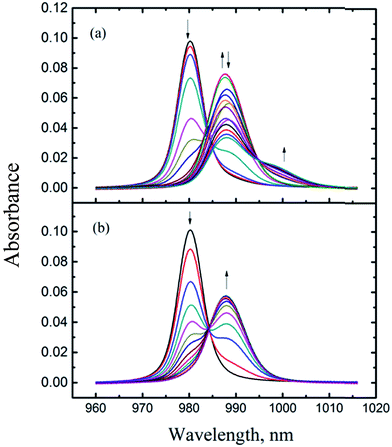Fig. 2 Spectrophotometric titrations of Np(V) with HEDTA at I = 1.0 mol L−1 NaClO4, t = 25 °C. Experimental conditions are provided in Table S1 of ESI:† (a) titration #4, (b) titration #1.

As mentioned in the Experimental section, the titrations were conducted in the N2-purged environment so that the entrance of carbon dioxide into the titration solution was minimized. Also, the base solutions for spectrophotometric titrations were prepared in the inert atmosphere chamber from the carbonate-free stock solutions. As a result, the presence of carbonate in the titration solutions was very limited. Even though a small amount of carbonate may exist in the solutions, the formation of Np(V) carbonate complexes would not occur under our experimental conditions due to the following reason. Carbonate forms Np(V) complexes only in the basis region (pH 7–12)26 where HEDTA forms much stronger complexes than carbonate, two orders of magnitude higher in the stability constant for a 1:1 complex.15,26 Under the experimental conditions of this work (see Table S1), the formation of Np(V)/carbonate complexes should be effectively suppressed because HEDTA was in excess amounts over Np(V) in the basic region.

Fig. 2(a) shows a titration covering a wide pCH (= −log[H+]) range (3.0 to 11) while Fig. 2(b) shows a titration where the highest pCH is 5.5. In Fig. 2(a), the absorption band of free NpO2+ at 980.2 nm was red-shifted as HEDTA was added. Approximately three isosbestic points could be identified at 984, 990, and 994 nm, suggesting four absorbing species (free NpO2+ and three Np(V)/HEDTA complexes) were present in the titration solutions. A number of models were tested to fit the spectra data in the whole range of pCH, including the model containing NpO2L2−, NpO2HL, and NpO2(OH)L3−,15 but none were satisfactory. In some cases, a fit could converge but result in unreasonably large, negative absorptivities for the NpO2(OH)L3− complex.

To better define the Np(V)/HEDTA complexes and obtain the stability constants, the titration data shown in Fig. 2(b) that cover a narrower range of pCH are processed first. Factor analysis of the spectra indicates the presence of three absorbing species and an excellent fit was achieved with the model including the formation of two Np(V)/HEDTA complexes: NpO2L2− and NpO2HL, represented by reactions (1) and (2). The fit yielded the stability constants of logβ101 (NpO2L2−) = 6.91 ± 0.06, and logβ1(11) (NpO2(HL)) = 4.28 ± 0.03.

 NpO2+ + L3− = NpO2L2− (1)
 NpO2+ + HL2− = NpO2HL− (2)

With the obtained stability constants of NpO2L2− and NpO2HL as known values, the spectra from multiple titrations covering the whole range of pCH (up to 11) were again fitted with models including an additional species that could become significant in basic solutions. In the fitting, the hydrolysis constants of the binary species, NpO2OH(aq) and NpO2(OH)2, were taken from the literature17 and held constant. The model including the NpO2(OH)L3− complex that was reported in the previous study15 did not converge. In contrast, the model including a dimeric species with the stoichiometry of (NpO2)2(OH)2L26− resulted in a good fit with reasonable molar absorptivity. The formation of (NpO2)2(OH)2L26− is represented by reaction (3), with an equilibrium constant of logβ2−22 ((NpO2)2(OH)2L26−) = −(4.93 ± 0.03).

 2NpO2+ + 2L3− + 2H2O = (NpO2)2(OH)2L26− + 2H+ (3)

The equilibrium constants of reactions (1)–(3) are summarized in Table 1. The deconvoluted molar absorptivities of the Np(V)/HEDTA complexes are shown in Fig. S1 of ESI. The equilibrium constants for reactions (1) and (2) from this work are in reasonable agreement with the values from previous studies, taking into consideration the difference in ionic strength. However, discrepancy exists in the model describing the data in the high pCH region: the data in the present study are best represented by the formation of (NpO2)2(OH)2L26− (reaction (3)), while the data from the previous study15 suggest the formation of NpO2(OH)L3−. Based on the EXAFS results from the present work, that are discussed in subsequent sections and a similar ternary dimeric U(VI)–OH–IDA complex suggested in the literature,27 we believe that at the high pCH area, Np(V) formed the ternary dimeric Np(V)–OH–HEDTA complex ((NpO2)2(OH)2L26−) with a similar configuration to that of the ternary dimeric U(VI)–OH–IDA complex,27 in which, each NpO2+ is coordinated with one HEDTA ligand and the two NpO2+ centers are bridged by two hydroxides.

Table 1 Thermodynamic parameters of the Np(V) complexation with HEDTA and other aminopolycarboxylate ligands at 25 °C in NaClO4. Legends: sp – spectrophotometry, cal – calorimetry, pot – potentiometry, sx – solvent extraction, p.w. – present work
Ligand Reaction I Method logβ ΔH, kJ mol−1 ΔS, J mol−1 K−1 Ref.
HEDTA NpO2+ + L3− = NpO2L2− 1.0 M sp/cal 6.91 ± 0.06 −(8.0 ± 2.0) 105 ± 7 p.w.
0.1 M sp 6.87 ± 0.11     15
6.6 m sx 7.25 ± 0.06     16
NpO2+ + HL2− = NpO2HL 1.0 M sp/cal 4.28 ± 0.03 −(2.2 ± 2.0) 75 ± 7 p.w.
0.1 M sp 4.06 ± 0.01     15
2NpO2+ + 2L3− + 2H2O = (NpO2)2(OH)2L26− + 2H+ 1.0 M sp −(4.93 ± 0.03)     p.w.
NpO2+ + L3− + H2O = NpO2(OH)L3− + H+ 0.1 M sp −(2.21 ± 0.09)     15
EDTA NpO2+ + L4− = NpO2L3− 0.1 M sp 7.33 ± 0.06     15
NpO2+ + HL3− = NpO2HL2− 1.0 M sp 4.89 ± 0.01     11
0.1 M sx 5.30 ± 0.08     15
NpO2+ + L4− + H2O = NpO2(OH)L4− + H+ 0.1 M sp −(2.27 ± 0.08)     15
NTA NpO2+ + L3− = NpO2L2− 1.0 M sp 6.30 ± 0.03     p.w.
0.1 M sp 6.80 ± 0.10     15
NpO2+ + HL2− = NpO2HL 1.0 M sp 1.79 ± 0.10     p.w.
0.1 M sp 1.77 ± 0.37     15
NpO2+ + L3− + H2O = NpO2(OH)L3− + H+ 0.1 M sp −(2.32 ± 0.11)     15
IDA NpO2+ + L2− = NpO2L 1.0 M pot/cal 5.85 ± 0.22 −(13.5 ± 1.8) 66.7 ± 6.5 13
0.1 M sp 6.27 ± 0.11     15
NpO2+ + HL = NpO2HL 0.1 M sp 1.35 ± 0.52     15

### 3.2 Enthalpy of complexation for Np(V)/HEDTA

Three calorimetric titrations were conducted with both Np(V) and HEDTA in the initial cup solution titrated with HClO4 or NaOH. The acid titrations (#1 and #2 in Table S2 of ESI) covered the low pCH range (8.5–3.5) so that the enthalpies of complexation for NpO2L2− and NpO2HL were determined. The base titration (#3 in Table S2 of ESI) extended the pCH range up to 10.5 with the objective of obtaining the enthalpy of complexation for (NpO2)2(OH)2L26−.

Fig. 3 shows the data of an acid titration. The reaction heats, in conjunction with the equilibrium constants of reactions (1) and (2) obtained by spectrophotometry in this work, and the protonation constants and enthalpy of protonation of HEDTA from the literature,28 were fitted to calculate the enthalpies of complexation for NpO2L2− and NpO2HL. As shown in Fig. 3 (lower figure), excellent fit was obtained. The calculated enthalpies of complexation for NpO2L2− and NpO2HL are listed in Table 1. In the pCH range of the acid titrations, the ternary (NpO2)2(OH)2L26− complex is negligible.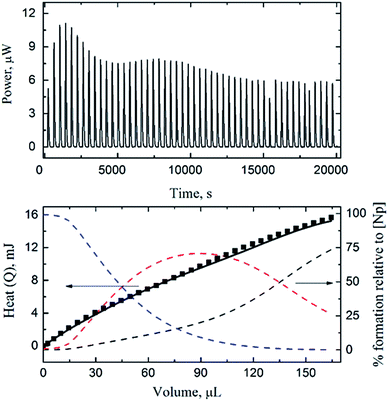Fig. 3 Calorimetric titration of NpO2+/HEDTA (t = 25 °C, I = 1.0 mol L−1 NaClO4). Upper figure: thermogram (dilution heat not corrected). Lower figure: observed (■) and calculated (-) total reaction heat (left y axis) and speciation of Np(V) (right y axis, dashed lines: NpO2+ (black), NpO2L2− (blue) and NpO2HL− (red)) as a function of titrant volume. Titration conditions are provided in Table S2 of ESI† (titration #1).

The data from the base titration (#3 in Table S2 of ESI) that covered a wide pCH range (up to 10.5) were fitted in two ways: a “global” fit for enthalpies of complexation for all three Np(V)/HEDTA complexes including the ternary (NpO2)2(OH)2L26− complex, or a restricted fit by holding the enthalpies of complexation for NpO2L2− and NpO2HL obtained from acid titrations as constants and fitting exclusively for (NpO2)2(OH)2L26−. None of the attempts were successful, this is most likely due to the combination of: (1) the maximum percentage of (NpO2)2(OH)2L26− in the titration was only ∼10%, probably too little for accurate calculation, and (2) unlike the spectrophotometric titrations, the calorimetric titration cell is in fact open to the atmospheric environment and the interference of carbon dioxide at high pCH cannot be ignored. As a result, only the enthalpies of complexation for NpO2L2− and NpO2HL are reported in Table 1. The corresponding entropies of complexation are also calculated from the enthalpies and free energies. The values of enthalpy and entropy of complexation, the first such data determined for the Np(V)/HEDTA system, indicate that both enthalpy and entropy favor the complexation, but the entropy is the dominant driving force for the complexation due to the chelating effect of the aminopolycarboxylic acids.

### 3.3 Thermodynamic trends and coordination modes in Np(V) complexes with aminopolycarboxylic acids

Table 1 lists the thermodynamic parameters of Np(V) complexes with HEDTA, EDTA, NTA and IDA at t = 25 °C and different ionic strengths. The ligands are structurally related, differing in the number of amino groups and/or carboxylate groups. Noticeably, HEDTA and EDTA possess two amino groups while NTA and IDA possess one amino group. Discussions on the thermodynamic trends could provide insights into the nature and possible coordination modes of the complexes of NpO2+ with the series of aminopolycarboxylic acids.

As shown in Fig. 4, excellent linear correlations are observed between the stability constants of ML and MHL complexes (where M stands for NpO2+) and the total basicity (i.e., ΣpKa) of the ligands, suggesting that the complexation of NpO2+ with the aminopolycarboxylic acids can be described by electrostatic interactions and the binding strength is directly related to the ligand basicity. In addition, the linearity of the correlation could imply that the coordination modes in the Np(V) complexes are probably similar for these ligands. However, as the following discussions suggest, while this could be true for the ML complexes of all aminopolycarboxylic acids, the coordination mode in the protonated complex (MHL) of the ligands with only one amino group (e.g., NTA and IDA) could be different from that of the ligands with more than one amino group (e.g., HEDTA and EDTA). Possible coordination modes in ML and MHL complexes are discussed below.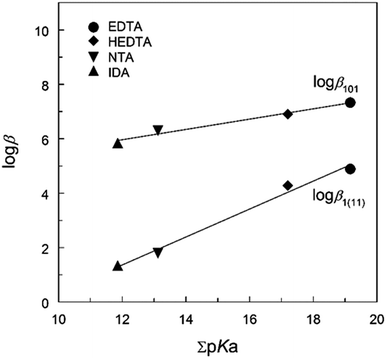Fig. 4 Stability constants vs. total ligand basicity (pKa) at t = 25 °C. logβ101 for NpO2L2− and logβ1(11) for NpO2(HL)−; all values at I = 1.0 mol L−1 NaClO4 except that of logβ1(11) for Np(V)/IDA at I = 0.1 mol L−1 NaClO4.
NpO2L(n−1)−. Due to the linear configuration of the NpO2+ ion, the ligands can only approach the center Np atom through the equatorial plane. A simpler trident ligand such as IDA could use all donor atoms, one N and two O atoms, to coordinate NpO2+,13,29 as shown in Fig. 5(a). However, due to steric restrictions, ligands with higher denticity such as HEDTA and EDTA could only use some of the donor atoms, at most one N and two O atoms, to coordinate NpO2+ through the equatorial plane, as shown in Fig. 5(b), leaving the other N atom and carboxylate groups not coordinated to the same Np center. Similar modes are suggested for the coordination of UO22+ with EDTA.30 This means that the local coordination environment of NpO2+ in the ML complexes with HEDTA, EDTA, NTA and IDA is similar as shown by Fig. 5(a) and (b).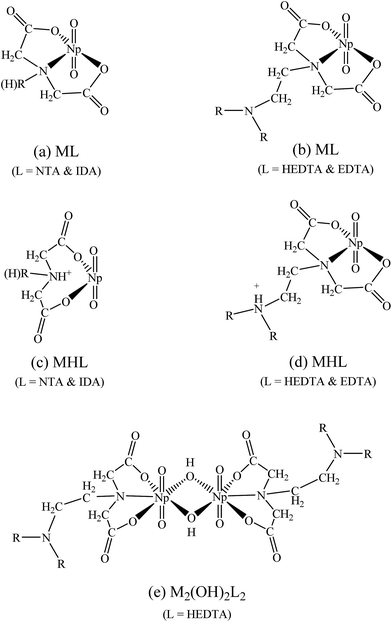Fig. 5 Possible coordination modes in the Np(V) complexes with aminopolycarboxylic acids. (a) ML complexes of NTA or IDA; (b) ML complexes of HEDTA or EDTA; (c) MHL complexes of NTA or IDA; (d) MHL complexes of HEDTA or EDTA; (e) M2(OH)2L2 of HEDTA.
NpO2(HL)(n−2)−. To help discuss the possible coordination modes in the protonated complexes of Np(V) with aminopolycarboxylic acids, additional spectrophotometric titrations with Np(V) and NTA were performed at I = 1.0 mol L−1 NaClO4. The absorption spectra and deconvoluted molar absorptivities of NpO2L2− and NpO2(HL) (where L3− stands for the fully deprotonated NTA ligand) are shown in Fig. S2 of ESI. The stability constants are listed in Table 1.

The formation of the protonated complex for HEDTA and NTA, NpO2(HL), is described by reaction (2). In general, the M(HL) complex is less stable than the ML complex for all aminopolycarboxylic acids shown in Table 1, which is understandable as the interactions are predominantly electrostatic in nature and ligand Ln has a higher negative charge than ligand HL(n−1)−. However, data in Table 1 indicate that the difference (logβ101 − logβ1(11)) for HEDTA and EDTA is much smaller than that for NTA and IDA: 2.4–2.6 for HEDTA and EDTA while 4.5–4.9 for NTA and IDA. Such contrast could probably help reveal the coordination modes in the protonated MHL complexes of NpO2+ with the aminopolycarboxylic acids as discussed below.

NTA and IDA possess only one amino N atom but HEDTA and EDTA possess two N atoms. Therefore it is reasonable to envisage that the protonation of the ML complexes with NTA or IDA occurs on the amino N atom so that it becomes weakly bonded to Np or does not bind Np at all, as shown in Fig. 5(c), which results in bidentate MHL complex (Fig. 5(c)) much weaker (more than 4 orders of magnitude in the stability constants) than the tridentate ML complex (Fig. 5(a)). In contrast, the protonation of the ML complexes with HEDTA and EDTA could probably occur on the amino N atom that is not directly bonded with Np, as shown in Fig. 5(d). In this case, the MHL complexes remain tridentate so that they are only slightly weaker (about 2 orders of magnitude in stability constants) than corresponding ML complexes with HEDTA and EDTA.

Comparison of the deconvoluted absorption spectra of the ML and MHL complexes for the Np(V)/HEDTA and Np(V)/NTA systems (Fig. 6) provides further rationalization of the above discussion. Data in Fig. 6 show that the positions of the absorption bands of the ML and MHL complexes are close for the Np(V)/HEDTA system (Fig. 6(a)), but drastically different for the Np(V)/NTA system (Fig. 6(b)). This observation is consistent with the above discussion that suggests the local coordination environment of Np in the MHL complex differs significantly from that in the ML complex for the NTA or IDA systems, but remains similar for the HEDTA or EDTA systems.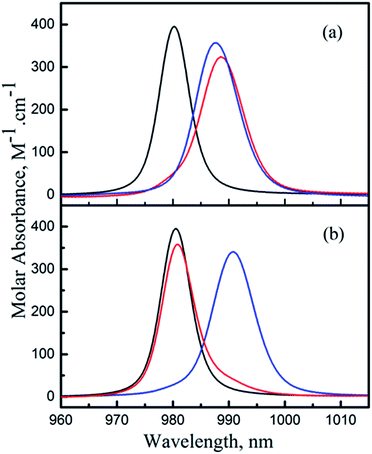Fig. 6 Resolved molar absorbance spectra of individual Np(V) species from the spectrophotometric titrations. (a) Np(V)/HEDTA, (b) Np(V)/NTA. NpO2+ (black), NpO2L2− (blue), NpO2HL− (red).

### 3.4 XAS results for structural analyses of Np(V)/HEDTA complexes

The raw k3-weighted Np LIII EXAFS spectra and their Fourier transform (FT) of three Np(V) solutions are presented in Fig. 7. The fit lines are also depicted in the figure. The structural fitting results as well as the Np(V) speciation of individual solutions are listed in Table 2.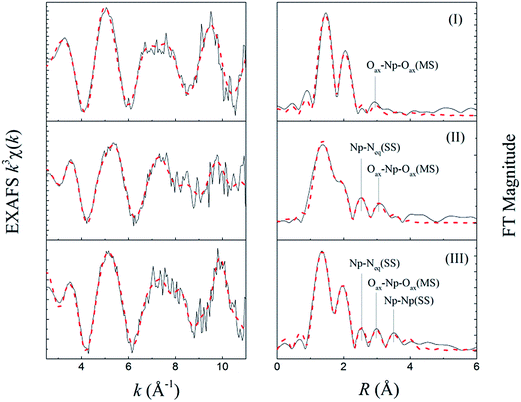Fig. 7 Np LIII EXAFS of three Np(V) solutions. (I) [Np] = 3.0 mmol L−1, pCH = 2.5; (II) [Np] = 1.0 mmol L−1, [HEDTA] = 2.3 mmol L−1, pCH = 9.4; (III) 2.0 mL, [Np] = 1.0 mmol L−1, [HEDTA] = 23 mmol L−1, pCH = 12. Black solid line – experimental, red dash line – fit.
Table 2 EXAFS fitting results of Np(V) species in solutions
Solutiona Shell Nb Rb (Å) σ2 Notice
a The Np(V) speciation (relevant to total [Np]) was calculated with the simulation program HySS2009 (ref. 35) using the complexation constants determined in this work.b Solution I: Np–Oax, R ± 0.01, N = 2.0 held constant; Np–Oeq, R ± 0.01, N ± 0.3. Solution II: Np–Oax, R ± 0.01, N = 2.0 held constant; Np–Oeq, R ± 0.02, N ± 0.3; Np–Neq, R ± 0.03, N ± 0.3. Solution III: Np–Oax, R ± 0.01, N = 2.0 held constant; Np–Oeq, R ± 0.01, N ± 0.2; Np–Neq, R ± 0.02, N ± 0.3; Np–Np, R ± 0.04, N ± 0.3.
I Np–Oax 2.0 1.82 0.0025 S02 = 0.85, ΔE0 = 5.56 eV, r = 0.005, χred2 = 13.0
Speciation: 100% NpO2+ Np–Oeq 4.6 2.51 0.0096
II Np–Oax 2.0 1.82 0.0046 S02 = 075, ΔE0 = 8.05 eV, r = 0.004, χred2 = 14.5
Speciation: 100% NpO2L2− (L = HEDTA) Np–Oeq 3.8 2.41 0.0114
Np–Neq 1.0 2.95 0.0047
III Np–Oax 2.0 1.82 0.0038 S02 = 0.77, ΔE0 = 9.01 eV, r = 0.005, χred2 = 15.1
Speciation: 11% NpO2L2−, 87% (NpO2)2(OH)2L26− Np–Oeq 3.7 2.40 0.0089
Np–Neq 1.0 2.93 0.0039
Np–Np 1.0 3.95 0.0046

For comparison, Table 3 provides selected bond distances of two structurally-relevant Np(V) compounds: tris(guanidinium)bis(n-methyliminodiacetato)-dioxo-neptunium(V)25 (Compound A) and catena-((μ6-oxalato)-(μ2-aqua)-(μ2-hydroxo)-neptunium(V)-di-sodium)31 (Compound B) reported in the literature. The crystal structures are depicted in Fig. S3 in ESI. The bond distances are selected from two motifs: tridentate binding structure of IDA to Np(V) in Compound A and double μ2-OH bridged Np–Np structure in Compound B.

Table 3 Selected bond distances of two Np(V) compounds
Compound A (Å) Compound B (Å) Notice
Np–Oax 1.84 1.85(1) Oxygen of dioxo group
Np–Ocarboxyl 2.538 2.51(1) Oxygen of carboxyl group
2.54(1)
Np–Ohydroxyl   2.40(1) Oxygen of hydroxyl group
2.43(1)
Np–Namine 2.732   Nitrogen of amine group
Np–Np   3.96

The FT in Fig. 7 represents a pseudo-radial distribution function, and the peaks are shifted to lower R values as a result of the phase-shifts associated with the absorber–scatter interactions (∼0.2–0.5 Å). The speciation results indicate that the dominant Np(V) species are NpO2+ (100%), NpO2L2− (100%) and (NpO2)2(OH)2L26− (87%) in solutions I, II and III, respectively, (L is denoted as fully deprotonated HEDTA). It is noteworthy that in solutions II and III, although the pH was high, the formation of Np(V) carbonate complexes could be ignored because of the following: (1) the solutions were carefully prepared from carbonate-free stock or working solutions, and well-sealed in the plastic vials so that the entrance of carbonate was minimized; (2) HEDTA was present in large excess over the amount of Np in both solutions ([HEDTA]/[Np] = 2.3/1.0 (II) and 23/1.0 (III)) so that the formation of carbonate-based No(V) species could be substantially suppressed as HEDTA is a much stronger complexant than carbonate. An excellent fit was achieved with the proposed coordination structures for those Np(V) species, the details of which are discussed below.

Solution I. An EXAFS analysis of solution I indicates that the dioxo Np(V) is surrounded by five oxygens (Oeq) in its equatorial plane at a distance of 2.51 Å (RNp–Oeq). The distance of dioxo oxygen (Oax) to Np(V) is 1.82 Å (RNp–Oax). Those results are in excellent agreement with the early work32,33 on the structure of hydrated neptunyl in aqueous solution.
Solution II. In solution II, the presence of HEDTA resulted in new EXAFS features. In the FT graph, a new peak that appeared at 2.5 Å was well fitted with the single scattering of one nitrogen atom (NNp–Neq = 1.0), which is believed to be the nitrogen of amine group of HEDTA. The peak at 2.0 Å, which is supposed to be a scattering shell of equatorial coordination atoms, was largely broadened. Various patterns, including double shells and a single shell were tested to fit the broadened peak at 2.0 Å, resulting in a single shell with NNp–Oeq = 3.8 and RNp–Oeq = 2.41 Å as the best fit.

It is noticeable that the resultant Debye–Waller factor of the Np–Oeq shell is quite large (σ2 = 0.0114, Table 2). The unusually large Debye–Waller factor suggests that this scattering shell is most likely to consist of two coordination shells, which could not be resolved due to the limited k range and the large noise of fluorescence signals. One shell forms by the coordinating HEDTA oxygens, the other by the hydration water oxygens. Considering the fact that the amine nitrogen participates in the coordination, it is reasonably believed that two carboxylate oxygens on the two sides of the amine group in the HEDTA molecules are among those 3.8 coordinating oxygens, forming a tridentate coordination mode in this Np(V)/HEDTA complex.

The resolved RNp–Oeq (2.41 Å) is appreciably smaller than that in solution I (2.51 Å). This implies that a bond distance of the carboxylate oxygens to Np(V) (Np–Ocarboxyl) in the Np(V)/HEDTA complex is shorter than that of the water oxygen to Np(V) (Np–Owater) in hydrated Np(V) species, which is often seen in the metal/carboxylate complexes in aqueous solutions. Considering the fact that the averaged value of Np–Ocarboxyl and Np–Owater distances is 2.41 Å (RNp–Oeq), the distance of Np–Ocarboxyl should be smaller than 2.41 Å.

A comparison of the Np–Ocarboxyl and Np–Neq distances to the corresponding bond distances in the Np(V)/IDA complex (Compound A) gains more insight into the coordination geometry in the 1:1 Np(V)/HEDTA complex. The comparison reveals that the Np–Ocarboxyl distance in the HEDTA complex (<2.41 Å) is much shorter than that in the IDA complex (2.54 Å, Table 3), and the resolved RNp–Neq (2.95 Å, Table 2) in the HEDTA complex is much longer than that in the Np(V)/IDA structure (2.73 Å, Table 3). These contrary variations might be attributed to the different steric hindrance of ligands in these two complexes. From the ligand perspective, both HEDTA and IDA hold the same tridentate coordination mode to Np(V). However, the coordination geometries of Np(V) in the two complexes are quite different. In Compound A, Np(V) holds six atoms (four oxygens and two nitrogens) in its equatorial coordination plane, generating relatively large steric hindrance and forcing the coordination atoms relatively far away from the central Np(V) atom. But, in the HEDTA complex, Np(V) holds five atoms (four oxygens and one nitrogen) with less steric hindrance in its equatorial plane, and two oxygens from the carboxylate groups, which are strongest in the binding strength among them, would bind to the Np(V) more closely and form the shorter Np–Ocarboxyl distance. In these two complexes, an amine group is in the middle of two coordinating carboxylates. The different Np–Ocarboxyl distances between the two complexes may play a role in their Np–Namine distances. As just discussed, the carboxylates of HEDTA in the HEDTA complex is quite close to Np(V), compared to those of IDA in the IDA complex. It is possible that the HEDTA amine may feel a steric force, and be expelled further away from the Np(V). As a result, the Np–Namine distance in the HEDTA complex becomes longer than that in the IDA complex. It is also noted that the IDA amine is secondary and the HEDTA amines are tertiary. Nitrogen atoms on the two types of amines may hold slightly different electron densities, which possibly affects the complexation strength. However, taking into account the fact that the amine nitrogens loosely bind to Np(V) in both complexes, the slight density variation hardly makes appreciable difference in the bond distance of Np–Namine. Therefore, we believe that the longer Np–Namine distance in the HEDTA complex is very likely caused by the steric effect as discussed above.

It is clear that the EXAFS result of solution II strongly supports the tridentate coordination mode of HEDTA in the 1:1 complex (NpO2L2−), i.e., two oxygens from two carboxylates and one nitrogen from the amine group participate in the coordination to Np(V). This is in agreement with the coordination mode yielded from the thermodynamic trends of Np(V) complexation with aminopolycarboxylic acids discussed in Section 3.3.

Solution III. The EXAFS of solution III holds the following features: (1) a new peak occurred at 3.5 Å; (2) the peak at 2.0 Å became much sharper, compared to that of solution II. The fit resulted in the following scattering shells: Np–Oeq (RNp–Oeq = 2.40, NNp–Oeq = 3.7), Np–Neq (RNp–Neq = 2.93, NNp–Neq = 1.0) and Np–Np (RNp–Np = 3.95, NNp–Np = 1.0). The presence of the Np–Np shell strongly demonstrates a formation of the dimeric Np(V) species, which has been proposed from the spectrophotometric data in the present work.

It is true that a structure of this dimeric complex cannot be decisively determined from the limited EXAFS data. However, referring to the reported dimeric U(VI) double-OH bridging structure27 and considering our determined stoichiometry, coordination numbers and bond distances for this ternary Np(V)–OH–HEDTA complex, we came to the suggestion that this complex may hold a similar double-OH bridging structure as described in Fig. 5(e). The details of our considerations are described below.

The coordination atoms of Np(V) under our proposed structure are composed of two oxygens from the HEDTA carboxylates, two oxygens from the two bridging hydroxyl groups and one nitrogen from the amine group. They are well matching the EXAFS resolved coordination numbers of the Np–Oeq shell (NNp–Oeq = 3.7, four oxygens), and the Np–Neq shell (NNp–Neq = 1.0, one nitrogen).

The bond distances of the Np–Oeq shell have received particular attention. As mentioned earlier, the peak at 2.0 Å, corresponding to the Np–Oeq shell, is quite sharp. This implies that in this scattering shell, all four coordinating oxygens might be located to Np(V) with a similar distance. In the proposed structure, four Np–O bonds likely fit to this feature. Hydroxide, unlike a water molecule, is a strong complexing agent so that the bond distance of metal to oxygen of hydroxide is shorter than that of metal to oxygen of water. For instance, in Compound B, the bond distance of Np–Ohydroxyl is ∼2.41 Å (Table 3), shorter than that of Np–Owater (2.51 Å, Table 2: Solution I) and close to the RNp–Oeq of solution III (2.40 Å, Table 2). Also, the early work34 has confirmed that the hydrolysis of metal cations could weaken the binding strength between metals and organic ligands, thereby increasing the metal–ligand bond length. From the EXAFS results of solutions I and II, we have understood that the bond distance of Np–Ocarboxyl in the complex NpO2L2− is smaller than 2.41 Å even though its exact value could not be resolved. The presence of hydroxide ions in the dimeric Np(V) complex could weaken this Np–Ocarboxyl bond and increase its distance. As a result, these four oxygens (two from the HEDTA carboxylates and two from the two hydroxyl groups) may form a coordination shell with a similar distance to the Np(V).

The Np–Np distance (3.95 Å, Table 2), resolved from the EXAFS, is in excellent agreement with the Np–Np distance (3.96 Å, Table 3) in the similar dimeric Np(V) motif in Compound B where two Np atoms are connected through double μ2-OH bridging (cf., Fig. S3 in ESI). This agreement further supports our proposed double-OH bridging structure.

In summary, the EXAFS results of solution III demonstrate that the ternary Np(V)–OH–HEDTA complex is a dimeric Np(V) species. It is very possible that two Np(V) atoms are connected through double-OH bridging. In the complex, each HEDTA maintains a tridentate coordination mode (Fig. 5(e)).

XANES spectra of three solutions are given in Fig. S4 in ESI. Those spectra present similar white lines and high energy shoulders, indicating that Np(V) in each solution holds the same dioxo and equatorial coordination mode. A special note is given to the inflection energy. Fig. S4 indicates that the inflection energy decreases from solution II (7.5 eV) to solution III (6.2 eV). This decrease can be attributed to a change in the coordination environments of Np(V) from the complex NpO2L2− to the (NpO2)2(OH)2L26−. From the above structural analysis, we understand that the significant change between these two complexes is the replacement of two water molecules by two hydroxide anions. In the complex (NpO2)2(OH)2L26−, the presence of two hydroxide anions in the inner coordination sphere of Np(V) could move the electron density of Np atom towards the ligands. As a result, the Np LIII edge energy decreases.

### 3.5 Speciation of Np(V) in the absence and presence of HEDTA

The speciation of Np(V) in a wide pCH range (2–12) in the absence and presence of HEDTA is shown in Fig. 8. In the absence of HEDTA, the first hydrolyzed species, NpO2(OH)(aq), starts to form as the pCH is increased to above 8, and the second hydrolyzed species, NpO2(OH)2, becomes dominant as pCH > 10. In the presence of HEDTA, the NpO2(OH)(aq) species does not form in the entire pCH range while NpO2(OH)2 only starts to form as pCH is increased to above 11 and never becomes dominant. In brief, at a molar ratio of CHEDTA/CNp = 5, HEDTA could effectively suppress the hydrolysis of Np(V) in aqueous solutions with pCH up to 12 (Fig. 8).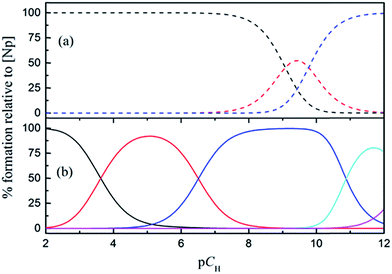Fig. 8 Speciation of Np(V) in absence (a) and presence (b) of HEDTA. CNp = 1.0 mmol L−1, CHEDTA = 5.0 mmol L−1. (a) NpO2+ (black), NpO2(OH)(aq) (red), NpO2(OH)2− (blue); (b) NpO2+ (black), NpO2HL− (red), NpO2L2− (blue), (NpO2)2(OH)2L26− (green), NpO2(OH)2− (magenta).

## 4 Summary

A model including three Np(V)/HEDTA complexes, NpO2HL, NpO2L2−, and (NpO2)2(OH)2L26−, is selected to describe the complexation of Np(V) with HEDTA. The stability constants of NpO2HL and NpO2L2−, determined at I = 1.0 mol L−1 NaClO4, are consistent with previous values determined at different ionic strengths. On the contrary to the previous model that includes NpO2(OH)L3−, the inclusion of the dimeric ternary species, (NpO2)2(OH)2L26−, results in the best fit of the data in the high pCH region. The EXAFS results have evidently demonstrated a formation of this dimeric tertiary Np(V)–OH–HEDTA complex.

The enthalpy and entropy of complexation for the NpO2HL and NpO2L2− indicate that the complexation of Np(V) with HEDTA is dominantly driven by entropy. The small but negative enthalpies of complexation suggest that the complexation would become weaker at higher temperatures.

The coordination mode of both NpO2HL and NpO2L2− is tridentate. Two oxygens from two carboxylic groups and one nitrogen from an amine group bind to Np(V). In the dimeric tertiary complex ((NpO2)2(OH)2L26−), two Np(V) atoms are bridged by double hydroxides and each HEDTA keeps the tridentate coordination mode.

## Acknowledgements

This work was supported by the Fuel Cycle Research and Development (FCR&D) Thermodynamics and Kinetics Program, Office of Nuclear Energy, the U.S. Department of Energy under Contract Number DE-AC02-05CH11231 at Lawrence Berkeley National Laboratory. L. R. Martin acknowledges the support from DOE NE FCR&D Thermodynamics and Kinetics program, under DOE Idaho Operations Office Contract DE-AC07-05ID14517 while preparing this manuscript. The EXAFS data were collected at Stanford Synchrotron Radiation Laboratory (SSRL). SSRL is a user facility operated for the U.S. DOE by Stanford University.

## References

1. R. G. Riley and J. M. Zachara, Chemical contaminants on DOS lands and selection of contaminant mixtures for subsurface science research, Pacific Northwest National laboratory, Richland, WA, 1992 Search PubMed.
2. A. P. Toste, T. J. Lechner-Fish, D. J. Hendren, R. D. Scheele and W. G. Richmond, J. Radioanal. Nucl. Chem., 1988, 123, 149–166 CrossRef CAS.
3. A. P. Toste, B. C. Osborn, K. J. Polach and T. J. Lechner-Fish, J. Radioanal. Nucl. Chem., 1995, 194, 25–34 CrossRef CAS.
4. A. A. Okemgbo, H. H. Hill, S. G. Metcalf and M. Bachelor, Anal. Chim. Acta, 1999, 396, 105–116 CrossRef CAS.
5. A. A. Okemgbo, H. H. Hill, S. G. Metcalf and M. Bachelor, J. Microcolumn Sep., 2000, 12, 48–56 CrossRef CAS.
6. A. Felmy and M. Mason, J. Solution Chem., 2003, 32, 283–300 CrossRef CAS.
7. A. Felmy, M. Mason and O. Qafoku, J. Solution Chem., 2003, 32, 301–318 CrossRef CAS.
8. S. G. Metcalf, Determination of EDTA and HEDTA in Hanford Defense Waste by High Performance Liquid Chromatography, Report RHO-SA-218, Rockwell Hanford Operations, Richland, WA, 1981 Search PubMed.
9. J. C. Braley, T. S. Grimes and K. L. Nash, Ind. Eng. Chem. Res., 2012, 51, 629–638 CrossRef.
10. E. N. Rizkalla, F. Nectoux, S. Dabos-Seignon and M. Pages, Radiochim. Acta, 1990, 51, 151–155 CrossRef CAS.
11. O. Tochiyama, C. Siregar and Y. Inoue, Radiochim. Acta, 1994, 66/67, 103–108 CAS.
12. O. S. Pokrovsky, M. G. Bronikowski, R. C. Moore and G. R. Choppin, Radiochim. Acta, 1998, 80, 23–29 CrossRef CAS.
13. G. Tian and L. Rao, Dalton Trans., 2010, 39, 9866–9871 RSC.
14. Y. Inoue and O. Tochiyama, Polyhedron, 1983, 2, 627–630 CrossRef CAS.
15. S. H. Eberle and U. Wede, J. Inorg. Nucl. Chem., 1970, 32, 109–117 CrossRef CAS.
16. P. Thakur and A. E. Van Luik, Radiochim. Acta, 2014, 102, 781–796 CrossRef CAS.
17. L. Rao, T. G. Srinivasan, A. Y. Garnov, P. Zanonato, P. Di Bernardo and A. Bismondo, Geochim. Cosmochim. Acta, 2004, 68, 4821–4830 CrossRef CAS.
18. G. Gran, Analyst, 1952, 77, 661–671 RSC.
19. P. Gans, A. Sabatini and A. Vacca, Talanta, 1996, 43, 1739–1753 CrossRef CAS PubMed.
20. P. Gans, A. Sabatini and A. Vacca, Ann. Chim., 1999, 89, 45–49 CAS.
21. J. J. Christensen, L. D. Hansen and R. M. Izatt, Handbook of Proton Ionization Heats and Related Thermodynamic Quantities, John Wiley & Sons, New York, 1976 Search PubMed.
22. P. Gans, A. Sabatini and A. Vacca, J. Solution Chem., 2008, 37, 467–476 CrossRef CAS.
23. B. Ravel and M. Newville, J. Synchrotron Radiat., 2005, 12, 537–541 CrossRef CAS PubMed.
24. S. I. Zabinsky, J. J. Rehr, A. Ankudinov, R. C. Albers and M. J. Eller, Phys. Rev. B: Condens. Matter Mater. Phys., 1995, 52, 2995 CrossRef CAS.
25. N. A. Budantseva, G. B. Andreev, A. M. Fedoseev and M. Y. Antipin, Dokl. Akad. Nauk SSSR, 2002, 384, 492 Search PubMed.
26. V. Neck, T. Fanghänel and J. I. Kim, Radiochim. Acta, 1997, 77, 167–175 CrossRef CAS.
27. J. Jiang, M. J. Sarsfield, J. C. Renshaw, F. R. Livens, D. Collison, J. M. Charnock, M. Helliwell and H. Eccles, Inorg. Chem., 2002, 41, 2799 CrossRef CAS PubMed.
28. X. Li, Z. Zhang, F. Endrizzi, L. R. Martin, S. Luo and L. Rao, J. Chem. Thermodyn., 2015, 85, 35–41 CrossRef CAS.
29. L. Rao and G. Tian, Symmetry, 2010, 2, 1–15 CrossRef CAS.
30. W. Hummel, G. Anderegg, I. Puigdomenech, L. Rao and O. Tochiyama, Chemical Thermodynamics of Compounds and Complexes of U, Np, Pu, Am, Tc, Zr, Ni and Se With Selected Organic Ligands, North Holland Elsevier Science Publishers B.V., Amsterdam, the Netherlands, 2005 Search PubMed.
31. A. C. Bean, E. Garcia, B. L. Scott and W. Runde, Inorg. Chem., 2004, 43, 6145–6147 CrossRef CAS PubMed.
32. P. G. Allen, J. J. Bucher, D. K. Shuh, N. M. Edelstein and T. Reich, Inorg. Chem., 1997, 36, 4676–4683 CrossRef CAS PubMed.
33. J. M. Combes, C. J. Chisholm-Brause, G. E. J. Brown, G. A. Parks, S. D. Conradson, P. G. Eller, I. R. Triay, D. E. Hobart and A. Miejer, Environ. Sci. Technol., 1992, 26, 376–382 CrossRef CAS.
34. F. Brulfert, S. Safi, A. Jeanson, E. Martinez-Baez, J. Roques, C. Berthomieu, P. L. Solari, S. Sauge-Merle and E. Simoni, Inorg. Chem., 2016, 55, 2728–2736 CrossRef CAS PubMed.
35. L. Alderighi, P. r. Gans, A. Ienco, D. Peters, A. Sabatini and A. Vacca, Coord. Chem. Rev., 1999, 184, 311–318 CrossRef CAS.

### Footnotes

Electronic supplementary information (ESI) available: Table S1: spectrophotometric titration conditions. Table S2: calorimetric titration conditions of Np(V)/HEDTA. Fig. S1: resolved molar absorptivities of individual Np(V) species for the Np(V)/HEDTA system. Fig. S2: spectrophotometric titrations of Np(V)/NTA complexation at I = 1.0 mol L−1 NaClO4, t = 25 °C. Fig. S3. crystal structures of two Np(V) compounds. Fig. S4. XANES spectra of three Np(V) solutions. See DOI: 10.1039/c6ra17818a
Present address: Nuclear Science and Isotope Technology Division, Oak Ridge National Laboratory, Oak Ridge, TN, 37831, USA.
 This journal is © The Royal Society of Chemistry 2016